The paper “Attention is all you need” from google propose a novel neural network architecture based on a self-attention mechanism that believe to be particularly well-suited for language understanding.

# Introduction

## Current Recurrent Neural Network

• Popular and successful for variable-length representations such as sequences (e.g. languages, time-series, etc)
• The gating models such as LSTM or GRU are for long-range error propagation.
• RNN are considered core of Seq2Seq with attention.
• The sequentiality prohibits parallelization within instances.
• Sequence-aligned states in RNN are wasteful.
• Hard to model hierarchical-alike domains such as languages.
• Long-range dependencies still tricky despite of gating models, so there are attempts to solve this by using CNN

## Current Convolutional Neural Network

• Trivial to parallelize (per layer)
• Fit intuition that most dependencies are local
• The problem of long-range dependencies of RNN has been achieved by using convolution.
• Path length between positions can be logarithmic when using dilated convolutions, left-padding for text. (autoregressive CNNs WaveNet, ByteNet)
• The complexity of $O(\log n)$ for ByteNet and $O(n)$ for CovS2S
• Long-range dependencies using CNN are referencing things by position while referencing by context makes much more sense.
• WaveNet and ByteNet architecture for example below.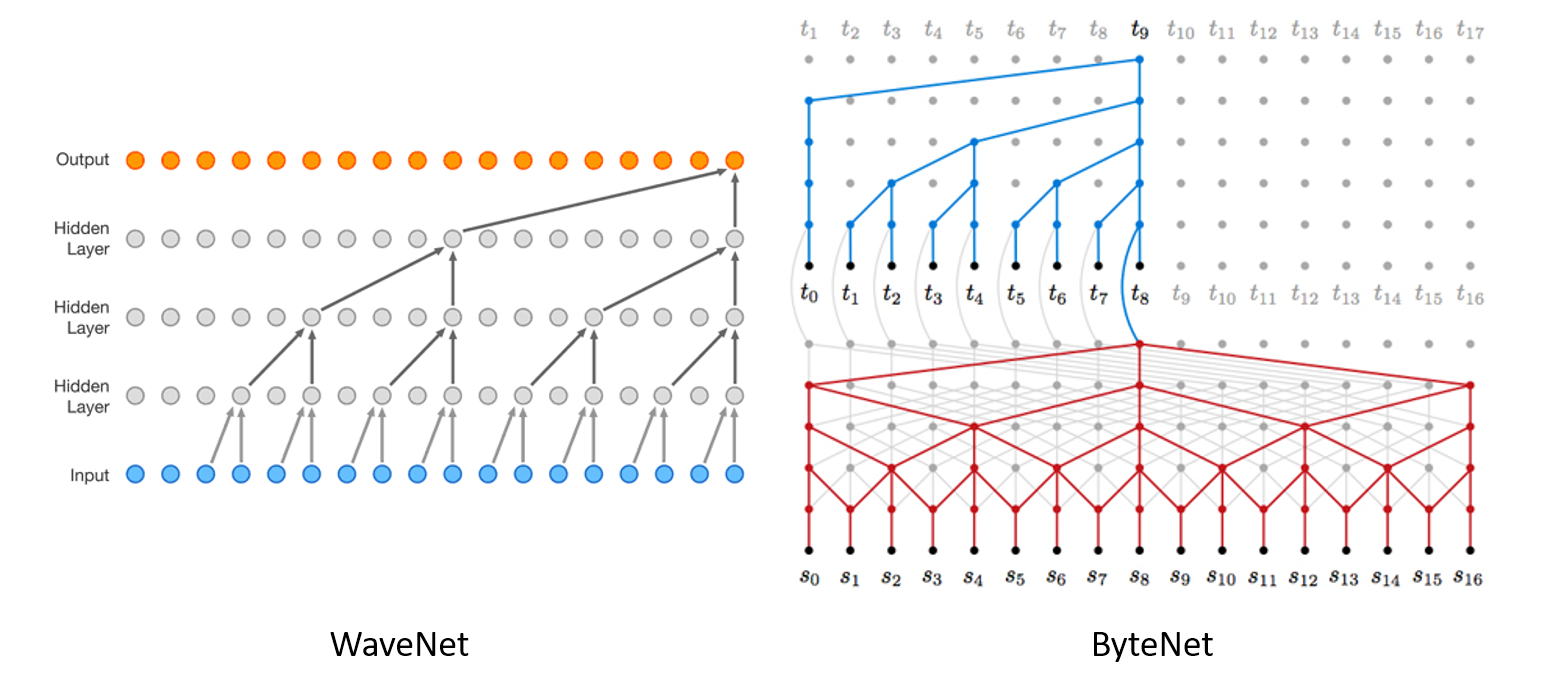# Attention

Attention between encoder and decoder is crucial in Neural Machine Translation (NMT). So, why not use (self-)attention for the representations?

## Self-Attention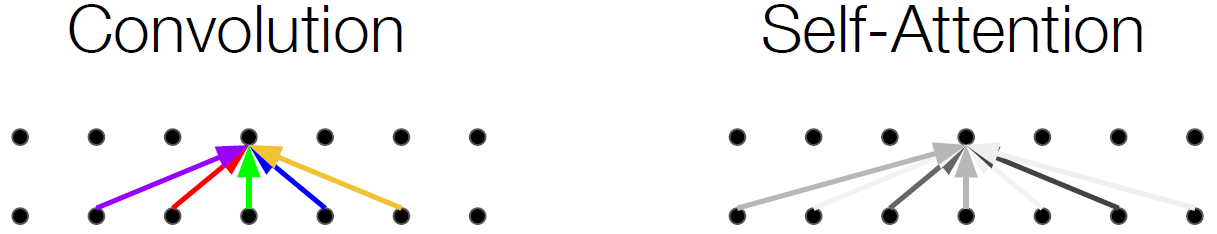• Convolution sees only positional things while self-attention looks at everything considering similarity.
• Sometimes called intra-attention
• Relating different positions of a single sequence in order to compute a representation of the sequence.
• Pairwise comparison between every two positions on signal
• Comparison is used to generate distribution for each positions to other positions in weighted average.

## Why Self-Attention?

1. Total computational complexity $O(1)$ by layer (v.s $O(n)$ in RNN)
2. Parallelizable computational capacity measured by minimum number of required sequential operations
3. Constant path length between long distance dependencies in the network
4. It is possible to generate a model in which self-attention can be more interpreted.

## Three Ways of Attention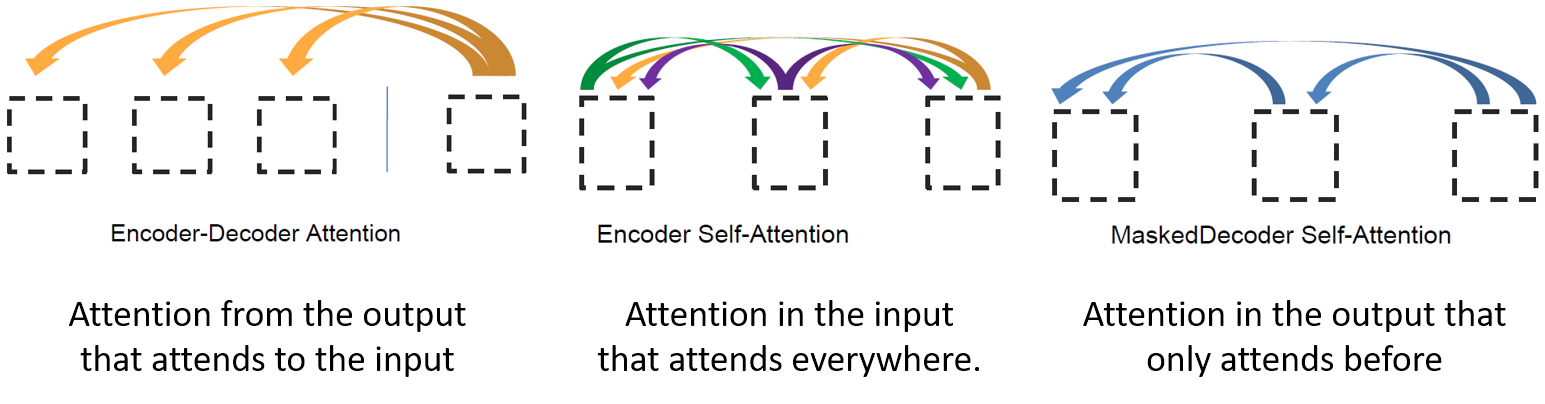# The Transformer

• Encoder-decoder structure of general neural sequence transduction models
• The encoder maps an input sequence of symbol representations $x$ to a sequence of continuous representation $z$.
• Given $z$, the decoder then generates an output sequence $y$ of symbols one element at a time.
• At each step the model is auto-regressive, consuming the previously generated symbols as additional input when generating the next.

Transformer follows this overall architecture using stacked self-attention and point-wise, fully connected layers for both the encoder and decoder.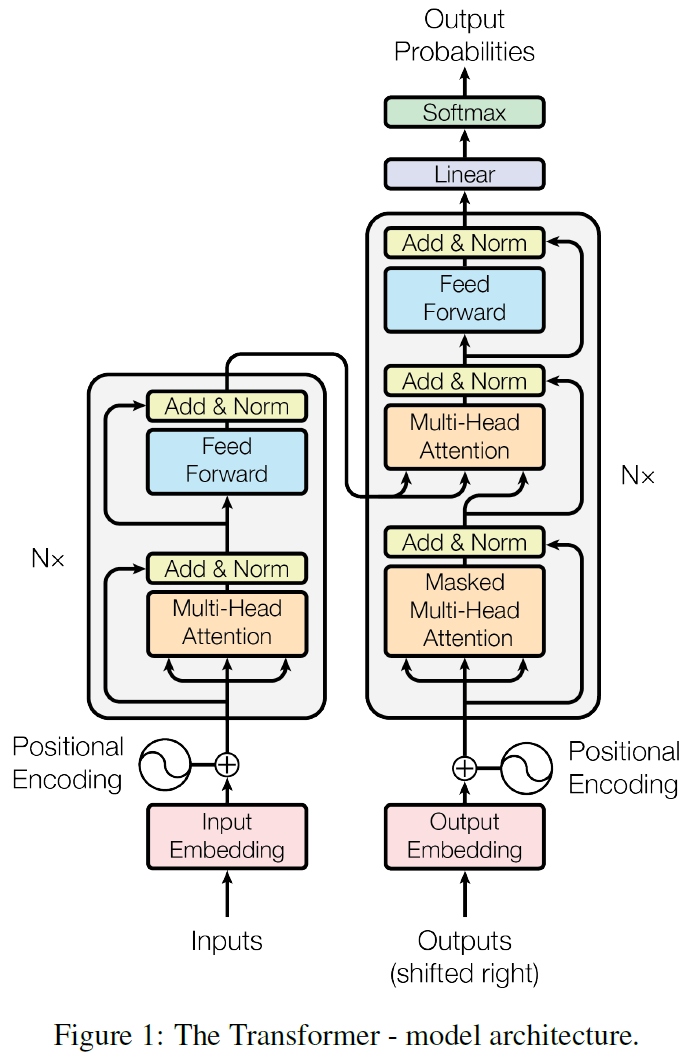• Encoder
• Composed of a stack of $N=6$ identical layers, each has two sub-layers
• The first is a multi-head self-attention mechanism, and the second is position-wise fully connected feed-forward network.
• Employ residual connection around each of the two sub-layers, followed by layer normalization
• Decoder
• Composed of a stack of $N=6$ identical layers, each has three sub-layers
• Two are the same with those of encoder, and a third sub-layer performs multi-head attention over the output of the encoder stack.
• Each sub-layer has residual connections, followed by layer normalization.
• Modify the self-attention sub-layer in the decoder stack to prevent positions from attending to subsequent positions.
• Example of how Transformer works## Scaled Dot-Product Attention

An attention function can be described as mapping a query and a set of key-value pairs to an output. The output is computed as a weighted sum of the values, where the weight assigned to each value is computed by a compatibility function of the query with the corresponding key.

The proposed scaled dot-product attention is described as below,

• $Q$: query vector (current word what I am operating on)
• $K$: key memory (find the most similar key)
• $V$: value memory (get the value corresponding the similar key)
• $d_k$: queries and keys of dimension
• $d_v$: values of dimension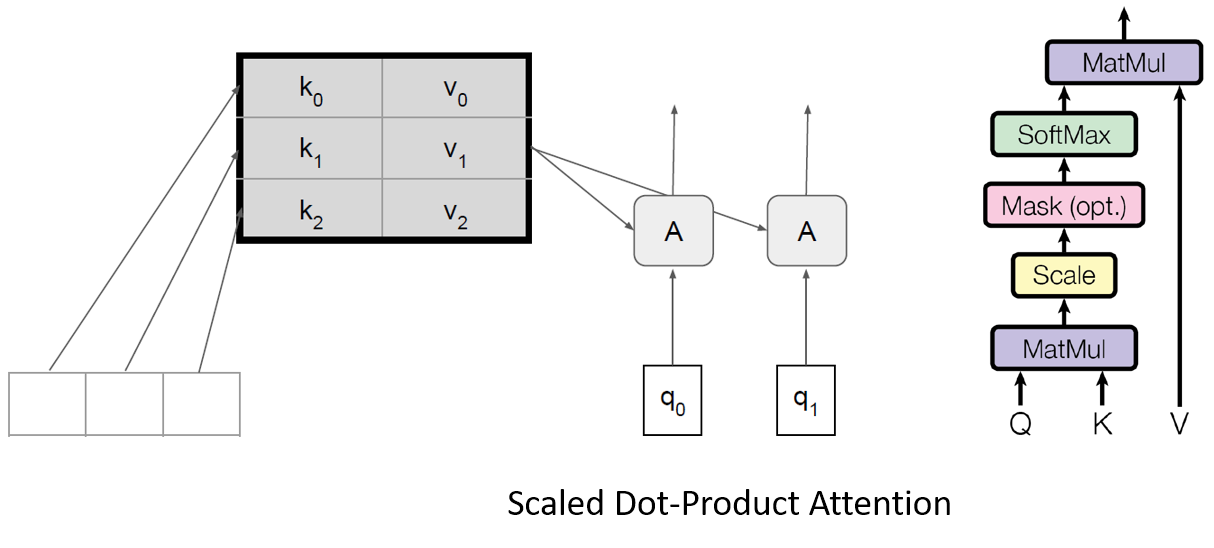There are two most commonly used attention functions: additive attention and dot-product attention. The Transformer uses dot-product attention and scaling factor of $\frac{1}{\sqrt{d_k}}$.

• Dot-product is quicker to calculate, it is more resistant to sparsity.
• It is easy to respond as in research.
• When $d_k$ is large, it is assumed that the gradient becomes smaller as the dot product becomes larger, so scaling by $\sqrt{d_k}$.
• Below table is time complexity comparison among attention, recurrent, and convolutional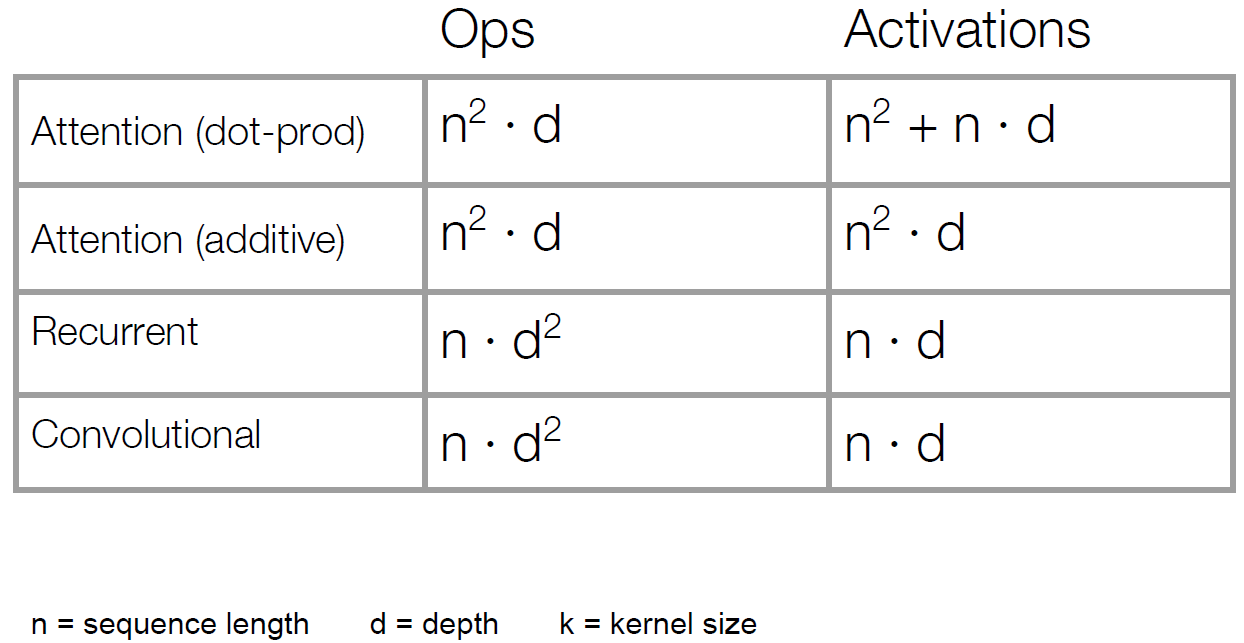• Almost every time, $d$ (usually 1000) is larger than $n$ (usually 20), so attention ($O(n^2d)$) is way faster than recurrent ($O(nd^2)$).

• What’s missing from Self-Attention?
• Convolution: a different linear transformation for each relative position. Allows you to distinguish what information came from where.
• Self-Attention: a weighted average
• Multiple attention layers (heads) in parallel (shown by different colors below figure)
• Each head uses different linear transformations.
• Different heads can learn different relationships.

Instead of performing a single attention function with $d_{model}$-dimensional keys, values and queries, it is beneficial to linearly project the queries, keys and values $h$ times with different, learned projections to $d_k$, $d_k$ and $d_v$ dimensions, respectively. On each of these projected versions of queries, keys and values we then perform the attention function in parallel, yielding $d_v$-dimensional output values.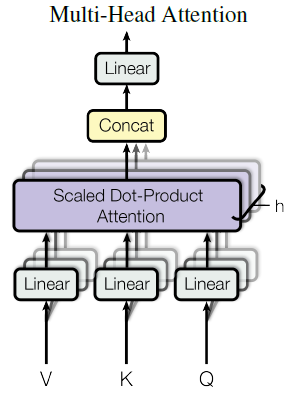Multi-head attention allows the model to jointly attend to information from different representation subspaces at different positions.

## Point-wise Feed-Forward Networks

• Feed-forward network which is applied to each position separately and identically.
• Consists of two linear transformations with ReLU activation in between.

## Positional Encoding

• Transmit location information since there is no recurrent or convolutional layer.
• There are many choices of positional encodings, in this work, sine and cosine functions of different frequencies are used.

# Experiments

• Data and Batch Processing
• WMT 2014 English - German Dataset and WMT 2014 English - French Language Data
• German: 4.5million sentence pairs and 37,000 tokens
• French: 36M sentences and split tokens into a 32,000 - sentence pairs are batched together with approximate sequence length
• Hardware and schedule
• One machine with 8 NVIDIA P100 GPUs
• Base model
• Approximately 0.4 seconds per each training step
• Total 100,000 step learning = 12 hours training
• Great model (big)
• Step time 1.0 seconds
• learning at 300,000 steps, 3.5 days

## Machine Translation Results: WMT-14

On the WMT 2014 English-to-German translation task, the big transformer model (Transformer (big) in Table 2) outperforms the best previously reported models (including ensembles) by more than 2.0 BLEU, establishing a new state-of-the-art BLEU score of 28.4.

On the WMT 2014 English-to-French translation task, our big model achieves a BLEU score of 41.0, outperforming all of the previously published single models, at less than 1/4 the training cost of the previous state-of-the-art model.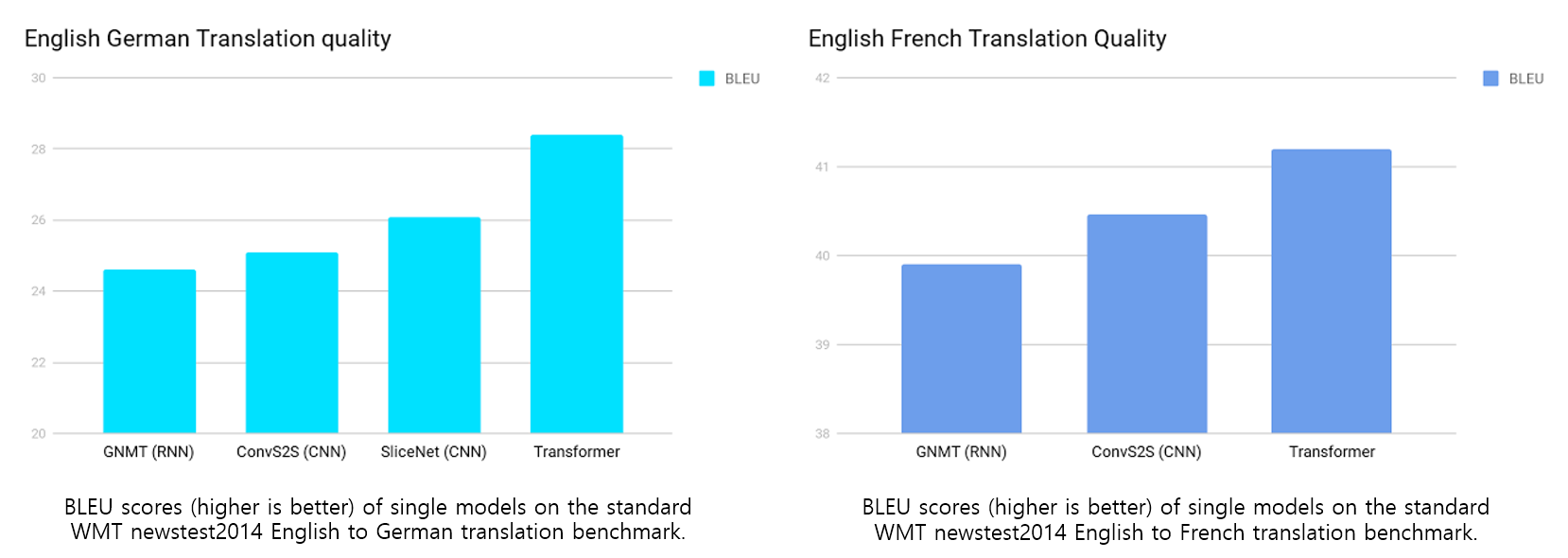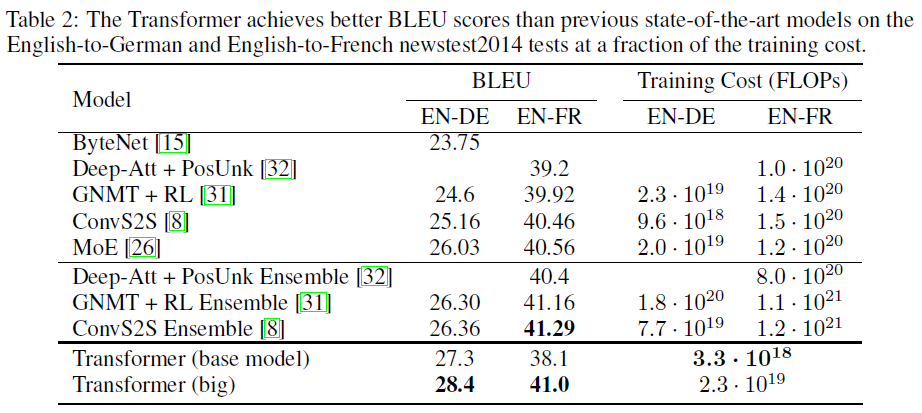## Variations on the Transformer

To evaluate the importance of different components of the Transformer, the authors varied the base model in different ways, measuring the change in performance on English-to-German translation on the development set, newstest2013.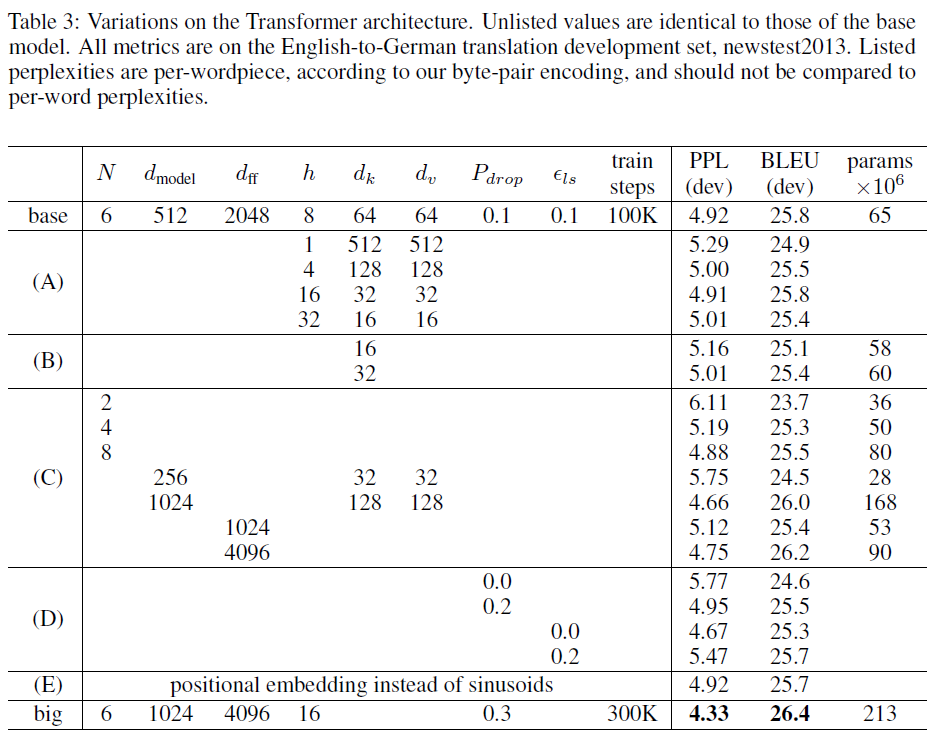• Google Research Blog: Transformer: A Novel Neural Network Architecture for Language Understanding [Link]
• Paper: Attention is all you need [Link]